#### Definitions and Notation

For a stratified clustered sample design, together with the sampling weights, the sample can be represented by an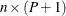matrix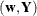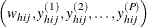where

•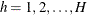is the stratum index

•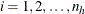is the cluster index within stratum h

•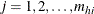is the unit index within cluster i of stratum h

•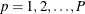is the analysis variable number, with a total of P variables

•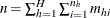is the total number of observations in the sample

•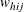denotes the sampling weight for unit j in cluster i of stratum h

•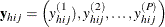are the observed values of the analysis variables for unit j in cluster i of stratum h, including both the values of numerical variables and the values of indicator variables for levels of categorical variables.

For a categorical variable C, let l denote the number of levels of C, and denote the level values as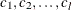. Let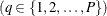be an indicator variable for the category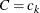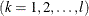with the observed value in unit j in cluster i of stratum h: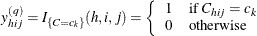Note that the indicator variable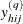is set to missing when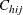is missing. Therefore, the total number of analysis variables, P, is the total number of numerical variables plus the total number of levels of all categorical variables.

The sampling rate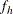for stratum h, which is used in Taylor series variance estimation, is the fraction of first-stage units (PSUs) selected for the sample. You can use the TOTAL= or RATE= option to input population totals or sampling rates. See the section Specification of Population Totals and Sampling Rates for details. If you input stratum totals, PROC SURVEYMEANS computesas the ratio of the stratum sample size to the stratum total. If you input stratum sampling rates, PROC SURVEYMEANS uses these values directly for. If you do not specify the TOTAL= or RATE= option, then the procedure assumes that the stratum sampling ratesare negligible, and a finite population correction is not used when computing variances. Replication methods specified by the VARMETHOD=BRR or the VARMETHOD=JACKKNIFE option do not use this finite population correction.